Courses

# Test: Simple Equations - 3

## 20 Questions MCQ Test Mathematics (Maths) Class 7 | Test: Simple Equations - 3

Description
This mock test of Test: Simple Equations - 3 for Class 7 helps you for every Class 7 entrance exam. This contains 20 Multiple Choice Questions for Class 7 Test: Simple Equations - 3 (mcq) to study with solutions a complete question bank. The solved questions answers in this Test: Simple Equations - 3 quiz give you a good mix of easy questions and tough questions. Class 7 students definitely take this Test: Simple Equations - 3 exercise for a better result in the exam. You can find other Test: Simple Equations - 3 extra questions, long questions & short questions for Class 7 on EduRev as well by searching above.
QUESTION: 1

### What is the value of 'x' in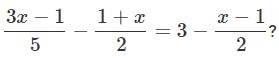Solution: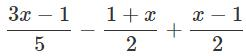= 3

⇒ 6x = 42 ⇒ x = 7

QUESTION: 2

### If 0.2(2x−1)−0.5(3x−1) = 0.4, what is the value of x?

Solution:

0.2(2x−1)−0.5(3x−1) = 0.4 ⇒ x = −1/11

QUESTION: 3

### In each of these figures the solution of an equation is given in brackets. Which of them is correct?

Solution:

Transposing 3 to the R.H.S. gives the value of m.

QUESTION: 4

Sunil wrote an equation as m/5 = 4. Ravi wrote a statement for Sunil's equation. Which of these is the statement of Ravi if he has written correctly?

Solution:
QUESTION: 5

In which of the following cases does an equality NOT hold?

Solution:
QUESTION: 6

What are the two steps involved in solving the equation 15x+4 = 26?

Solution:
QUESTION: 7

Which of the following equations can be constructed with x = 2?

Solution:
QUESTION: 8

7 subtracted from 5/2 of a number results in 23. What is the number?

Solution:

Let the number be x.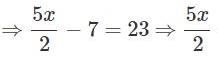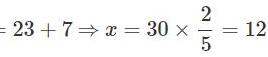QUESTION: 9

In a coconut grove, (x+2) trees yield 60 coconuts per year, x trees yield 120 coconuts per year and (x−2) trees yield 180 coconuts per year. If the average yield per year per tree is 100, find x.

Solution:

The average yield par tree per year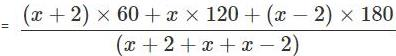⇒ 60x = 240 ⇒ x = 4

QUESTION: 10

4 is added to a number and the sum is multiplied by 5. If 20 is subtracted from the product and the difference is divided by 8, the result is equal to 10. Find the number.

Solution:

Let the number be x.
Then according to the problem,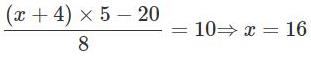QUESTION: 11

A number is 3 less than two times the other. If their sum is increased by 7, the result is 37. Find the numbers.

Solution:

Let the other number be x. So one number is 2x-3.when 7 is added their sum becomes 37
x+2x-3+7=37
3x+4=37
3x=33
x=11
One number=22-3=19

QUESTION: 12

1/2 is subtracted from a number and the difference is multiplied by 4. If 25 is added to the product and the sum is divided by 3, the result is equal to 10. Find the number.

Solution:

Let the number be x

According to the question

½ is subtracted from x which will give x – ½

And the difference is multiplied with 4 [x – 1/2] * 4 to give 5

[x – 1/2] * 4 = 5

4x – ½*4 = 5

4x -2 = 5

4x = 5 + 2

x = 7/4

QUESTION: 13

The present age of A is twice that of B. 30  years from now, age of A will be 11/2 times that of B. Find the present ages (in years) of A and B respectively.

Solution:

Let the present ages of A and B be 2x years and x years.
After 30 years, their ages will be
A = (2x+30) years
B = (x+30)  years So, 2x+30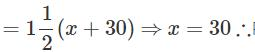Present age of B = 30 years ⇒  Present age of  A = 2×30 = 60  years

QUESTION: 14

A person travelled 5/8 th of the distance by  train, 1/4 th by bus and the remaining 15 km by boat. Find the total distance travelled by him.

Solution:

Let the total distance be = x

Distance travelled by train = 5/8 of x

Distance travelled by bus = 1/4 of x

Distance travelled by boat = 15 km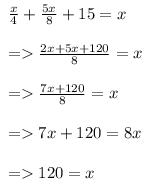Therefore, total distance = 120 km.

QUESTION: 15

The total cost of three prizes is Rs.2550. If the value of second prize is 3/4 th of the first and the value of 3rd prize is 1/2of the second prize, find the value of first prize.

Solution:

Let the value of the first prize be x.
Value of second prize =Value of third prize =The total cost of three prizes =Rs.2550QUESTION: 16

Which of the following is an equation?

Solution:
QUESTION: 17

Which of the following is an algebraic expression for the statement "The sum of 3x and 11 is 32."?

Solution:
QUESTION: 18

Choose the statement that best describes the equation 1/4 m = 10.

Solution:
QUESTION: 19

Vinay's father is 44 years old. If he is 5 years older than thrice Vinay's age, which of these equations gives, the age of Vinay's father?

Solution:
QUESTION: 20

Which of the following statements is false?

Solution: